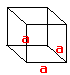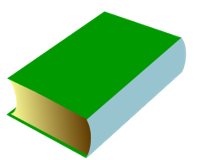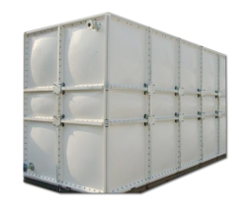Volume of Cube homework Help at TutorEye

## Top Questions

t schedule 3 people for the day my % scheduled is 78.57% My question is how did the computer come up with the 78.57%?
View More

I would love to get a written solution. I did the other proof tree that you can see in the attaached files. So its supposed to look like that Thank You.
View More

xtbook. i really need in in half an hour
View More

## Volume of Cube:The Cube is considered as a 3D- shape with 6 faces, 8 vertices and 12 edges.  The volume of the cube can be determined as the cube of each side.

Volume of a Cube = a x a x a = a3

## Volume of Cube Sample Questions:

Question 1: Calculate the volume of the cube with a side of 3.5 cm

(a) 12.5 cm3

(b) 75 cm3

(c) 42.9 cm3

(d) 3.50 cm3

Explanation: Plug the value of side in the volume of cube formula.

Question 2: What will be the side of the cube with a volume of 3375 cm3?

(a) 15 cm

(b) 1.5 cm

(c) 150 cm

(d) None

Explanation: Plug the value of volume in the volume of cube formula.

Question 3: What is the relation between diagonal length and volume of a cube?

(a) volume of cube = [√3×(diagonal)3]/6

(b) volume of cube = [√3×(diagonal)3]/4.

(c) volume of cube = [√3×(diagonal)3]/3

(d) volume of cube = [√3×(diagonal)3]/9

Explanation: Volume of Cube = [√3×(diagonal)3]/9

Question 4: Which of the following statements is incorrect?

(a) Cube has 6 faces

(b) Cube has 12 faces

(c) Cube has 8 vertices

(d) Cube has 12 edges

Explanation: Cube has 6 faces, 8 vertices and 12 edges.

Question 5: If the edge of a Rubik’s cube is 0.65 m, what will be the volume of the Rubik’s cube?

(a) 2.75 m3

(b) 0.275 m3

(c) 0.0275 m3

(d) 0.375 m3

Explanation: Plug the value of side in the volume of cube formula.

Question 6: A cubical box of external dimensions 110 mm each, also open at the top. If the cubical box is made of 5 mm wood thick, what will be the volume of the cube?

(a) 105 x 106 mm3

(b) 1050 x 106 mm3

(c) 10.5 x 106 mm3

(d) 1.05 x 106 mm3

Explanation: Length / Width = 110 – 5x 2 = 100 mm

Height = (110 – 5) mm = 105 mm

Question 7: Calculate the volume, in cm of a rectangular prism with following data:

Length = 1.65 cm

Width =  5.6 mm

Height =8.2 m

(a) 105 x 106 mm3

(b) 1050 x 106 mm3

(c) 10.5 x 106 mm3

(d) 1.05 x 106 mm3

Explanation: Plug the value of sides in the volume of cube formula.

Question 8: Identify the object with cube shape.

(a)(b)(c)(d)Explanation: Cube has 6 faces, 8 vertices and 12 edges.

Question 9: How many dice can fit in this box of 12 cm×10 cm×11 cm,  if the dice are cubes with sides of length 2 cm ?

(a) 110

(b) 150

(c) 101

(d) 11

Explanation: 6 dice = on the edge of the box measuring 12 cm
5 dice = on the edge of the box measuring 10 cm

Question 10: Calculate the volume of the cube with a diagonal of 9 cm.

(a) 140 cm3

(b) 1.40 cm3

(c) 0.140 cm3

(d) 14 cm3

Explanation: Volume of the cube = (√3 × d3)/9

## Volume of Cube Frequently Asked Questions:

Question 1: How to find the volume of a cube?

A cube is a solid three-dimensional object with six square faces.The total space occupied by an object is defined as its volume.

Volume, V = (side)3 cubic units.

Question 2: The side length, s, of a cube is x – 2y. if v = s3, what is the volume of the cube?Question 3: The side length, s, of a cube is 3x + 2y. if v = s3, what is the volume of the cube?